## Example. Estimating the risk of tumor in a group of rats¶

In :
%matplotlib inline
import numpy as np
import matplotlib.pyplot as plt
import pymc3 as pm
import seaborn as sns
import theano.tensor as tt

plt.style.use('seaborn-darkgrid')
plt.rc('font', size=12)

%config Inline.figure_formats = ['retina']

In :
rat_tumor = np.loadtxt('data/rat_tumor_data.txt', skiprows=3)

In :
# If you can use bash

# Rat tumor data from Tarone (1982).  Data from Table 5.1 of Bayesian Data Analysis.

y N
0 20
0 20
0 20
0 20
0 20
0 20
0 20


As you can see, the first column of rat_tumor is $y$ and the second column is $N$.

In :
print('The shape of rat_tumor is {}'.format(rat_tumor.shape))

The shape of rat_tumor is (71, 2)


We construct the model.

In :
with pm.Model() as model_1:

alpha = pm.Uniform('alpha', lower=0, upper=10)
beta = pm.Uniform('beta', lower=0, upper=25)

theta = pm.Beta('theta', alpha, beta, shape=rat_tumor.shape)

y = pm.Binomial('y', n=rat_tumor[:, 1], p=theta, observed=rat_tumor[:, 0])

In :
model_1.check_test_point()

Out:
alpha_interval__     -1.39
beta_interval__      -1.39
theta_logodds__     -21.37
y                  -256.01
Name: Log-probability of test_point, dtype: float64
In :
pm.model_to_graphviz(model_1)

Out:
In :
with model_1:
trace_1 = pm.sample(draws=5_000, tune=10_000)

Auto-assigning NUTS sampler...
Multiprocess sampling (4 chains in 4 jobs)
NUTS: [theta, beta, alpha]
Sampling 4 chains: 100%|██████████| 60000/60000 [01:26<00:00, 695.44draws/s]
The number of effective samples is smaller than 25% for some parameters.

In :
pm.traceplot(trace_1, varnames=['alpha', 'beta']);

/home/rosgori/anaconda3/lib/python3.6/site-packages/matplotlib/axes/_base.py:3455: MatplotlibDeprecationWarning:
The ymin argument was deprecated in Matplotlib 3.0 and will be removed in 3.2. Use bottom instead.
alternative='bottom', obj_type='argument')
/home/rosgori/anaconda3/lib/python3.6/site-packages/matplotlib/axes/_base.py:3455: MatplotlibDeprecationWarning:
The ymin argument was deprecated in Matplotlib 3.0 and will be removed in 3.2. Use bottom instead.
alternative='bottom', obj_type='argument')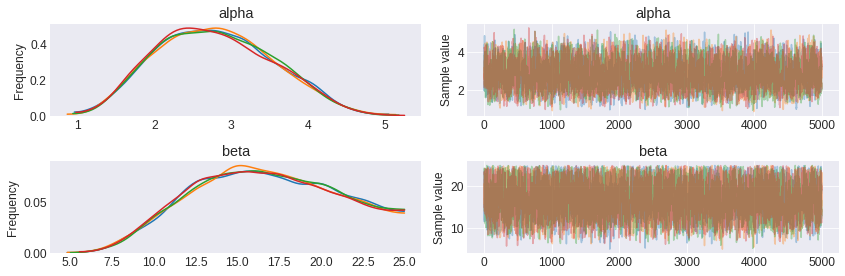In :
pm.summary(trace_1, varnames=['alpha', 'beta'])

Out:
mean sd mc_error hpd_2.5 hpd_97.5 n_eff Rhat
alpha 2.792588 0.743189 0.013334 1.436732 4.184145 2956.149772 1.000011
beta 16.730428 4.254348 0.072454 9.882307 24.998128 3307.434924 1.000008

The posterior plot of alpha and beta.

In :
pm.plot_posterior(trace_1, varnames=['alpha', 'beta']);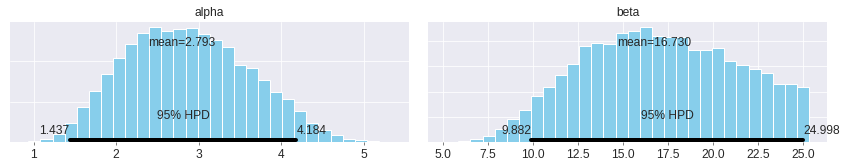As you can see, I have used a uniform distribution for alpha and beta. You could say that it is a regular prior, not good, not bad, but at the end disappointing. So let's try to improve the priors.

The following is taken from here. According to the book, the prior for $\alpha$ and $\beta$ is

$$p(\alpha, \beta) \propto (\alpha + \beta) ^{-5/2}$$

In order to use this prior, you have to define the logarithm of $p(\alpha, \beta)$ and use it in pm.Potential, look here.

In :
def logp_ab(value):
"""
Prior density for this problem
"""
return tt.log(tt.pow(tt.sum(value), -5/2))

with pm.Model() as model_10:
# Uninformative prior for alpha and beta
ab = pm.HalfFlat('ab', shape=2, testval=np.asarray([1., 1.]))
pm.Potential('p(a, b)', logp_ab(ab))

# Our alpha and beta
alpha = pm.Deterministic('alpha', ab)
beta = pm.Deterministic('beta', ab)

theta = pm.Beta('theta', alpha=ab, beta=ab, shape=rat_tumor.shape)

p = pm.Binomial('y', p=theta, observed=rat_tumor[:,0], n=rat_tumor[:,1])

In :
with model_10:
trace_10 = pm.sample(draws=5_000, tune=5_000)

Auto-assigning NUTS sampler...
Multiprocess sampling (4 chains in 4 jobs)
NUTS: [theta, ab]
Sampling 4 chains: 100%|██████████| 40000/40000 [00:52<00:00, 767.32draws/s]
There were 18 divergences after tuning. Increase target_accept or reparameterize.
There were 10 divergences after tuning. Increase target_accept or reparameterize.
There were 4 divergences after tuning. Increase target_accept or reparameterize.
There were 4 divergences after tuning. Increase target_accept or reparameterize.
The number of effective samples is smaller than 25% for some parameters.

In :
pm.traceplot(trace_10, varnames=['alpha', 'beta']);

/home/rosgori/anaconda3/lib/python3.6/site-packages/matplotlib/axes/_base.py:3455: MatplotlibDeprecationWarning:
The ymin argument was deprecated in Matplotlib 3.0 and will be removed in 3.2. Use bottom instead.
alternative='bottom', obj_type='argument')
/home/rosgori/anaconda3/lib/python3.6/site-packages/matplotlib/axes/_base.py:3455: MatplotlibDeprecationWarning:
The ymin argument was deprecated in Matplotlib 3.0 and will be removed in 3.2. Use bottom instead.
alternative='bottom', obj_type='argument')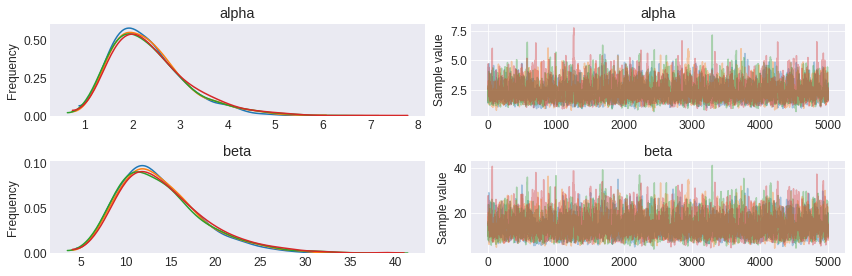In :
pm.summary(trace_10, varnames=['alpha', 'beta'])

Out:
mean sd mc_error hpd_2.5 hpd_97.5 n_eff Rhat
alpha 2.360068 0.808869 0.015864 1.046701 4.004286 3048.905773 1.001841
beta 14.057333 4.807157 0.093237 6.204920 23.892376 3222.664424 1.001573
In :
pm.plot_posterior(trace_10, varnames=['alpha', 'beta']);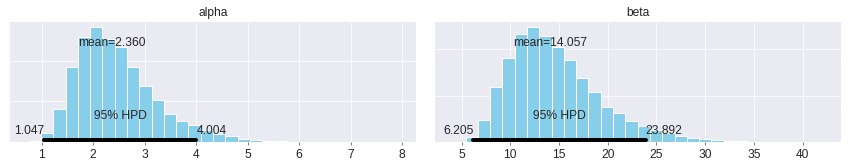Better, don't you think? And they are closer to the value $(\alpha, \beta) = (2.4, 14.0)$ showed in the book.

The next plots are a contour plot and a scatter plot.

In :
begi = 4_000

alpha_trunc = trace_10['beta'][begi: begi + 1000]
beta_trunc = trace_10['alpha'][begi: begi + 1000]

fig, ax = plt.subplots(figsize=(8, 7))
sns.kdeplot(np.log(alpha_trunc /  beta_trunc), np.log(alpha_trunc + beta_trunc),
cmap=plt.cm.viridis, n_levels=11, ax=ax)
ax.set_xlim(1.5, 2.1)
ax.set_ylim(1.7, 3.75)
ax.set_xlabel('log(alpha / beta)')
ax.set_ylabel('log(alpha + beta)');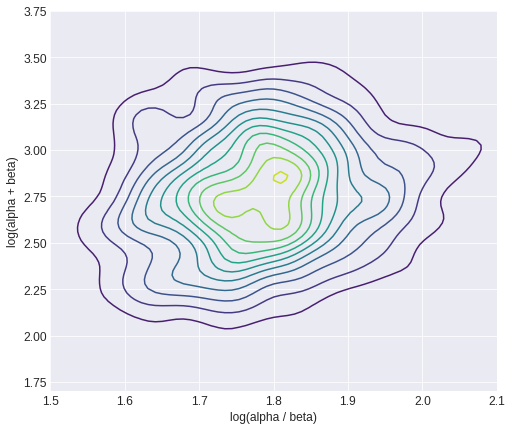In :
g = sns.jointplot(np.log(alpha_trunc /  beta_trunc), np.log(alpha_trunc + beta_trunc),
alpha=0.8, color='C5', kind='hex', height=8)
g.set_axis_labels('log(alpha / beta)', 'log(alpha + beta)');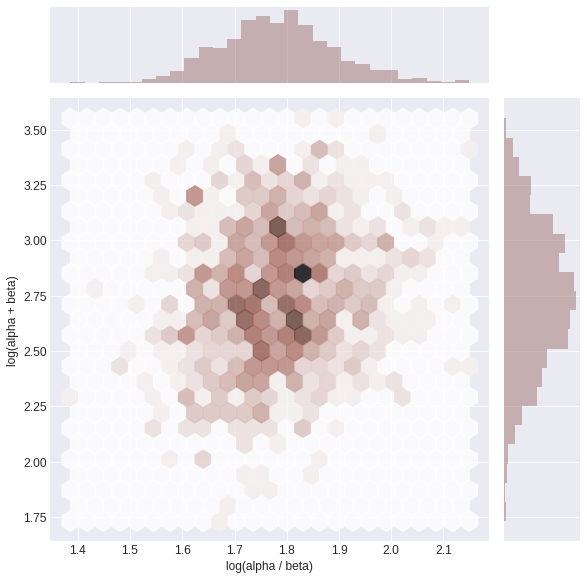Now, we draw 1000 samples for theta from the posterior distribution.

In :
post_theta = pm.sample_ppc(trace_10, samples=1000, model=model_10, vars=[theta], progressbar=False)


Posterior medians and 95% highest posterior density of rat tumor rates, $\theta_j$ (plotted vs. observed tumor rates $y_j / n_j$).

In :
median_theta = []

for i in range(post_theta['theta'].shape):
median_theta.append(np.median(post_theta['theta'][:, i]))

error_up = []
error_down = []

for i in range(post_theta['theta'].shape):
a, b = pm.hpd(post_theta['theta'][:, i], alpha=0.05)
error_up.append(b)
error_down.append(a)

plt.figure(figsize=(10,8))
plt.errorbar(rat_tumor[:, 0] / rat_tumor[:, 1], median_theta, fmt='o',
yerr=[error_down, error_up], ecolor='C5', markerfacecolor='k', mec='k',
errorevery=1)
plt.plot(np.linspace(0, .4, 10), np.linspace(0, .4, 10),
color='k')
plt.xlabel('Observed rate, y(i) / N(i)', fontsize=14)
plt.ylabel('95% hightest posterior density for each theta', fontsize=14);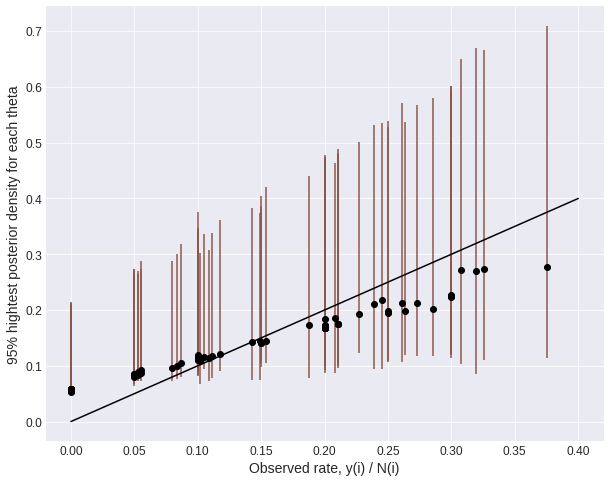## Example: parallel experiments in eight schools¶

Data of the eight schools model.

In :
yy = np.array([28.,  8., -3.,  7., -1.,  1., 18., 12.])
sigma = np.array([15., 10., 16., 11.,  9., 11., 10., 18.])

In :
with pm.Model() as model_2:
mu = pm.Uniform('mu', lower=0, upper=30)
tau = pm.Uniform('tau', lower=0, upper=30)
theta = pm.Normal('theta', mu=mu, sd=tau, shape=yy.shape)
obs = pm.Normal('obs', mu=theta, sd=sigma, observed=yy)

In :
pm.model_to_graphviz(model_2)

Out:
In :
with model_2:
trace_2 = pm.sample(draws=15_000, tune=30_000, nuts_kwargs={'target_accept':0.99})

Auto-assigning NUTS sampler...
Multiprocess sampling (4 chains in 4 jobs)
NUTS: [theta, tau, mu]
Sampling 4 chains: 100%|██████████| 180000/180000 [16:11<00:00, 185.24draws/s]
There were 99 divergences after tuning. Increase target_accept or reparameterize.
The acceptance probability does not match the target. It is 0.9589009742029759, but should be close to 0.99. Try to increase the number of tuning steps.
There were 129 divergences after tuning. Increase target_accept or reparameterize.
There were 15 divergences after tuning. Increase target_accept or reparameterize.
There were 45 divergences after tuning. Increase target_accept or reparameterize.
The number of effective samples is smaller than 10% for some parameters.

In :
pm.traceplot(trace_2, varnames=['mu', 'tau']);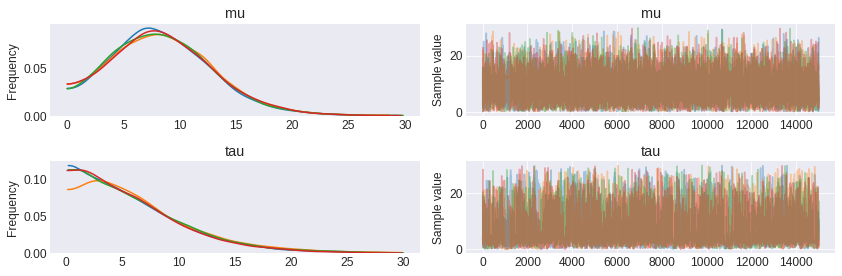In :
pm.summary(trace_2)

Out:
mean sd mc_error hpd_2.5 hpd_97.5 n_eff Rhat
theta__0 11.683407 8.072628 0.079508 -2.364170 29.202658 9540.086988 1.000255
theta__1 8.241418 6.072992 0.042111 -3.844689 20.508132 18621.837948 1.000002
theta__2 6.644003 7.352964 0.058526 -9.293521 20.750359 18105.525260 1.000052
theta__3 8.034519 6.311785 0.043290 -4.543239 20.898769 18477.560143 0.999985
theta__4 5.535042 6.087216 0.064419 -7.261684 16.908282 11157.549331 1.000168
theta__5 6.588537 6.431270 0.052744 -7.068402 18.973000 16525.202110 1.000116
theta__6 10.932158 6.576583 0.060985 -0.679374 25.058582 10407.796898 1.000057
theta__7 8.862579 7.519571 0.048141 -5.562598 25.369402 19120.224809 1.000053
mu 8.534240 4.530875 0.041987 0.000951 16.493687 9770.491536 1.000061
tau 6.315065 4.984469 0.092827 0.151026 16.171447 3002.260439 1.001085

The posterior plot of tau

In :
pm.plot_posterior(trace_2, varnames=['tau']);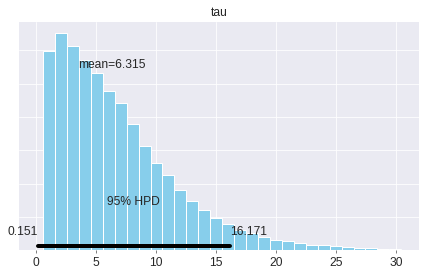We draw 1000 samples of tau and theta from the posterior distribution.

In :
with model_2:
post_trace_sample = pm.sample_ppc(trace_2, samples=1_000, vars=[tau, theta])

100%|██████████| 1000/1000 [00:00<00:00, 12923.44it/s]

In :
def partition_mean(ll, part):
"""
It takes two arguments, a list of pairs of points, (x, y), and the
length of the partition. It returns two lists, the first is a
list of means for each partiton on x and the second is a list of means
for each partition on y.

Returns:
mean_x::list
mean of each partition on x
mean_obs::list
mean of each partition on y
"""
mean_x = []
mean_obs = []

for i in range(0, len(ll), part):
ll_temp = ll[i: i + part]
x_temp = []
y_temp = []
for j in range(len(ll_temp)):
x_temp.append(ll_temp[j])
y_temp.append(ll_temp[j])
mean_x.append(np.mean(x_temp))
mean_obs.append(np.mean(y_temp))

return mean_x, mean_obs

def partition_std(ll, part):
"""
It takes two arguments, a list of pairs of points, (x, y), and the
length of the partition. It returns two lists, the first is a
list of means for each partiton on x and the second is a list of
standard deviation for each partition on y.

Returns:
mean_x::list
mean of each partition on x
mean_obs::list
standard deviation of each partition on y
"""
mean_x = []
mean_obs = []

for i in range(0, len(ll), part):
ll_temp = ll[i: i + part]
x_temp = []
y_temp = []
for j in range(len(ll_temp)):
x_temp.append(ll_temp[j])
y_temp.append(ll_temp[j])
mean_x.append(np.mean(x_temp))
mean_obs.append(np.std(y_temp, ddof=1))

return mean_x, mean_obs


This plot is for the conditional posterior means of treatment effects, $E(\theta_j \mid \tau, y)$, as functions of the between-school standard deviation $\tau$, for the educational testing example.

In :
plt.figure(figsize=(13, 7))

for i, z in zip(range(post_trace_sample['theta'].shape),
['A', 'B', 'C', 'D', 'E', 'F', 'G', 'H']):
tau_post = post_trace_sample['tau']
obs_post = post_trace_sample['theta'][:, i]

# It is necessary to sort the list of pairs of points
ll = sorted(list(zip(tau_post, obs_post)))
x, y = partition_mean(ll, 100)
plt.plot(x, y, label=z)

plt.xlabel('tau', fontsize=14)
plt.ylabel('Estimated treatment effects', fontsize=14)
plt.legend();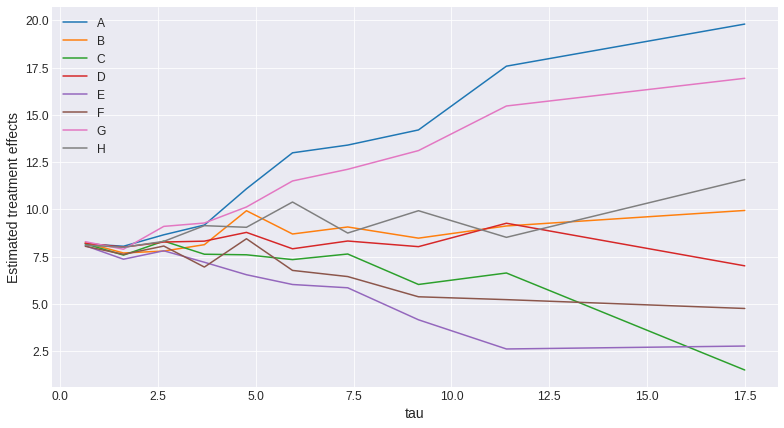This plot is for the conditional posterior standard deviations of treatment effects, $\textrm{sd}\,(\theta_j \mid \tau, y)$, as functions of the between-school standard deviation $\tau$, for the educational testing example.

In :
plt.figure(figsize=(13, 7))

for i, z in zip(range(post_trace_sample['theta'].shape),
['A', 'B', 'C', 'D', 'E', 'F', 'G', 'H']):
tau_post = post_trace_sample['tau']
obs_post = post_trace_sample['theta'][:, i]

# It is necessary to sort the list of pairs of points
ll = sorted(list(zip(tau_post, obs_post)))
x, y = partition_std(ll, 100)
plt.plot(x, y, label=z)

plt.xlabel('tau', fontsize=14)
plt.ylabel('Posterior standard deviations', fontsize=14)
plt.legend();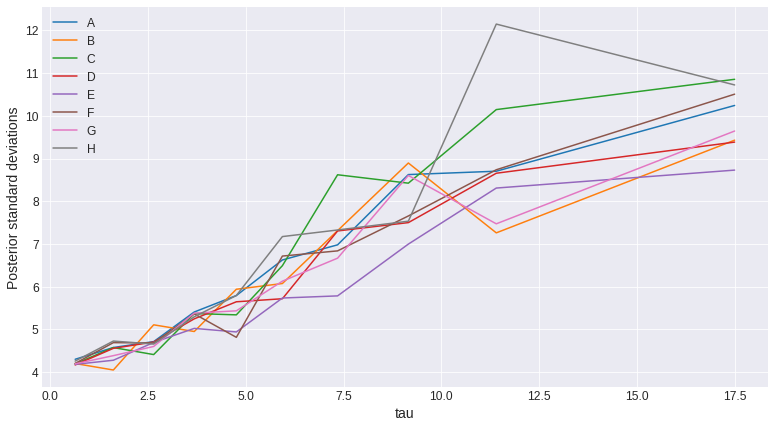We draw 200 samples of obs.

In :
with model_2:
post_obs = pm.sample_ppc(trace_2, samples=200, vars=[obs])

100%|██████████| 200/200 [00:00<00:00, 3248.50it/s]


Reproducing the Table 5.3

In :
print('{:^1} {:^50}'.format('School', 'Posterior quantiles'))
print('{:^7} {:^10} {:^10} {:^10} {:^10} {:^10}'.format(' ', '2.5%', '25%', 'median', '75%', '97.5%'))
print('-' * 70)

for i, z in zip(range(post_obs['obs'].shape),
['A', 'B', 'C', 'D', 'E', 'F', 'G', 'H']):

a1 = np.percentile(post_obs['obs'][:, i], 2.5)
a2 = np.percentile(post_obs['obs'][:, i], 25)
a3 = np.percentile(post_obs['obs'][:, i], 50)
a4 = np.percentile(post_obs['obs'][:, i], 75)
a5 = np.percentile(post_obs['obs'][:, i], 97.5)
print('{:^7} {:^10.0f} {:^10.0f} {:^10.0f} {:^10.0f} {:^10.0f}'.format(z, a1, a2, a3, a4, a5))

School                Posterior quantiles
2.5%       25%       median      75%       97.5%
----------------------------------------------------------------------
A       -18         -1         8          18         43
B       -15         2          8          15         29
C       -33         -4         6          19         43
D       -18         -1         6          15         31
E       -15         -1         5          12         23
F       -21         -4         6          14         30
G       -11         2          9          18         37
H       -23         -3         8          19         42

In :
plt.figure(figsize=(10, 6))
_, _, _ = plt.hist(post_trace_sample['theta'][:, 0], color='C5', bins=25, edgecolor='w')
plt.xlabel('Effect in school A', fontsize=14);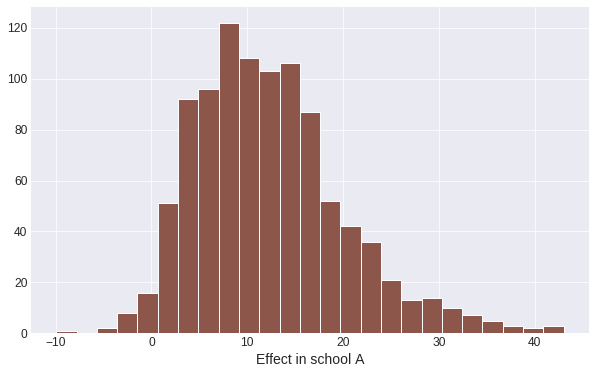In :
max_theta = []

for i in range(200):
with model_2:
post_theta = pm.sample_ppc(trace_2, samples=200, vars=[theta], progressbar=False)
max_theta.append(np.max(post_theta['theta'][:, :]))

In :
plt.figure(figsize=(10, 6))
_, _, _ = plt.hist(max_theta, color='C5', bins=25, edgecolor='w')
plt.xlabel('Largest effect', fontsize=14);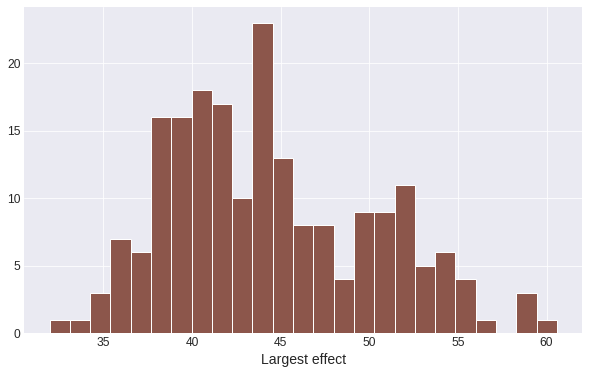In :
np.mean(np.array(max_theta) >= 28.4)

Out:
1.0

Ok, so the results are not quite good, I mean, there are a lot of discrepancies and the differences are notorious when you compare the two histograms previously showed with the Figure 5.8 (even more if you compare it with Table 5.3). Yes, the question is: Why does this happen? Is the algorithm behind pymc3 the reason of all this? I would say that priors are really bad and the geometry behind the model is nasty. More info here: https://docs.pymc.io/notebooks/Diagnosing_biased_Inference_with_Divergences.html

Changing the prior of tau_sq to inv-gamma(1, 1).

In :
with pm.Model() as model_3:
mu = pm.Uniform('mu', lower=0, upper=30)
tau_sq = pm.InverseGamma('tau_sq', 1, 1)
theta = pm.Normal('theta', mu=mu, sd=tau_sq, shape=yy.shape)
obs = pm.Normal('obs', mu=theta, sd=sigma, observed=yy)

In :
model_3.check_test_point()

Out:
mu_interval__    -1.39
tau_sq_log__     -1.31
theta            -1.81
obs             -31.29
Name: Log-probability of test_point, dtype: float64
In :
with model_3:
trace_3 = pm.sample(draws=5_000, tune=15_000)

Auto-assigning NUTS sampler...
Multiprocess sampling (4 chains in 4 jobs)
NUTS: [theta, tau_sq, mu]
Sampling 4 chains: 100%|██████████| 80000/80000 [01:36<00:00, 828.29draws/s]
There were 342 divergences after tuning. Increase target_accept or reparameterize.
There were 632 divergences after tuning. Increase target_accept or reparameterize.
The acceptance probability does not match the target. It is 0.6556958887998723, but should be close to 0.8. Try to increase the number of tuning steps.
There were 304 divergences after tuning. Increase target_accept or reparameterize.
The acceptance probability does not match the target. It is 0.7055707909541307, but should be close to 0.8. Try to increase the number of tuning steps.
There were 1070 divergences after tuning. Increase target_accept or reparameterize.
The acceptance probability does not match the target. It is 0.5612203902993772, but should be close to 0.8. Try to increase the number of tuning steps.
The estimated number of effective samples is smaller than 200 for some parameters.

In :
pm.traceplot(trace_3, varnames=['mu', 'tau_sq']);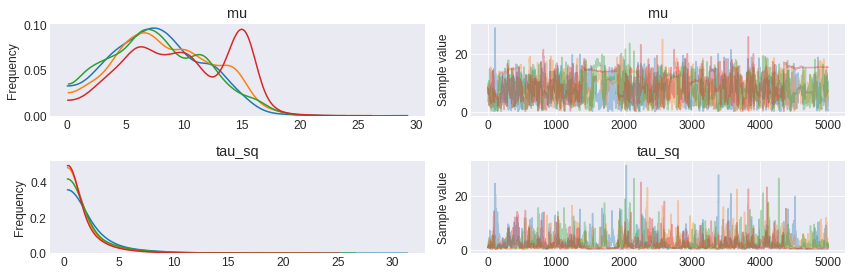In :
pm.plot_posterior(trace_3, varnames=['tau_sq']);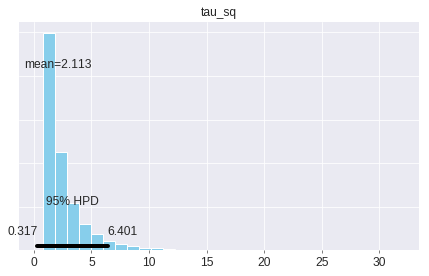Although this plot is similar to the Figure 5.9, they are not the same.

Changing the prior of tau_sq to inv-gamma(0.001, 0.001).